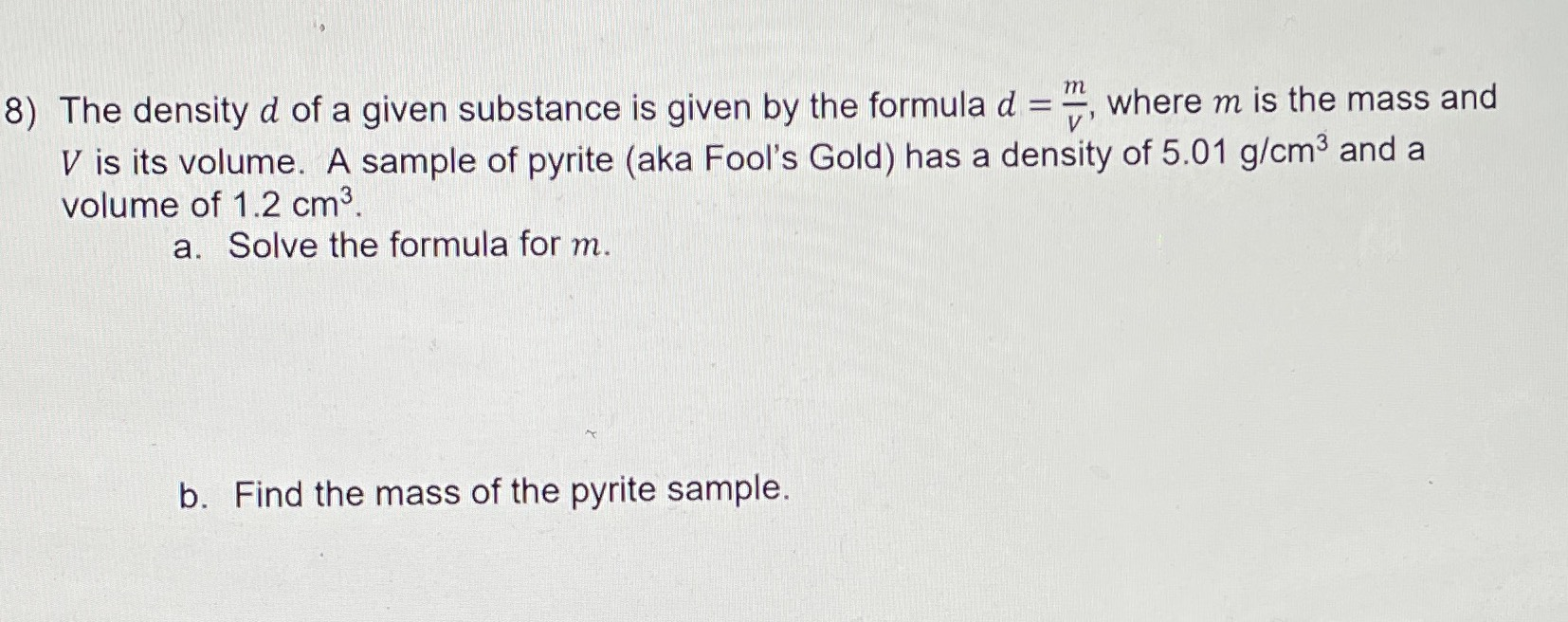### ¿Todavía tienes preguntas de matemáticas?

Pregunte a nuestros tutores expertos
Algebra
Pregunta8) The density $$d$$ of a given substance is given by the formula $$d = \frac { m } { V } ,$$ where $$m$$ is the mass and

$$V$$ is its volume. A sample of pyrite (aka Fool's Gold) has a density of $$5.01 g / cm ^ { 3 }$$ and a volume of $$1.2 cm ^ { 3 }$$ . a. Solve the formula for $$m$$ . b. Find the mass of the pyrite sample.